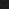JavaPerspective.com  >   Beginner Tutorials  >   3. Java Basics  >   3.6. Java operators examples  >   3.6.4. Bitwise operators examples

3.6.4. Bitwise operators examples
Last updated: 23 January 2013.

Let's write a simple method called bitwiseOperatorsTest to illustrate the uses of bitwise operators:

public void bitwiseOperatorsTest(){

byte a = 5; // the binary representation of a is 00000101

byte b = 6; // the binary representation of b is 00000110

System.out.println(a & b);       // the binary result is 00000100. The output is 4

System.out.println(a | b);    // the binary result is 00000111. The output is 7

System.out.println(a ^ b);    // the binary result is 00000011. The output is 3

System.out.println(~ a);    // the binary result is 11111010. The output is -6

System.out.println(a << 1);    // the binary result is 00001010. The output is 10

System.out.println(b >> 1);    // the binary result is 00000011. The output is 3

System.out.println(b >>> 1);// the binary result is 00000011. The output is 3

}

You are here :  JavaPerspective.com  >   Beginner Tutorials  >   3. Java Basics  >   3.6. Java operators examples  >   3.6.4. Bitwise operators examples
Next tutorial :  JavaPerspective.com  >   Beginner Tutorials  >   3. Java Basics  >   3.7. Java method calling